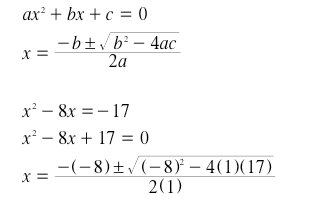SEARCH HOMEMath Central Quandaries & QueriesQuestion from Drew, a student: A solution of x^2-8x=-17 is -4 or -4+I or 4 or 4+iHi Drew,

Since this is a quadratic equation we know that it can have at most two solutions of the four. When you take the -17 to the other side of the equation it is not simple to factor. The easiest way to find which solution(s) are correct is substituting each value into the given equation. If you are not given any solutions, use the quadratic equation formula:Keep in mind that any negative under the square root will result in a complex root (containing an "i").
For more information on using the quadratic formula check out : http://mathcentral.uregina.ca/QQ/database/QQ.09.06/s/aranxa1.html

JaniceMath Central is supported by the University of Regina and The Pacific Institute for the Mathematical Sciences.